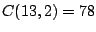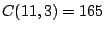# Enumerating Omaha Hands: Part II

Brian Alspach

Poker Digest, Vol. 5, No. 15, July 12 - 25, 2002

In the first article of this series on enumerating Omaha hands, we determined that there are 463,563,500,400 distinct Omaha hands. We then found that 411,293,904 of these hands give the player a straight flush. So a player should make a straight flush about once every 1,127 hands when playing Omaha.

We now want to continue with the determination of the numbers of other types of high hands. (We'll save low hands for later.) The framework for carrying out the enumeration is simple: Take all possible subsets of nine cards chosen from a 52-card deck and then partition each subset of nine cards into all possible player hands and boards. There areways of choosing nine cards from 52. There are 126 ways to partition nine cards into four player cards.

Of course, the size of the preceding numbers forces us to organize our attack in such a way the computations are feasible. The first step is a stratification of the subsets of nine cards. The following table presents the numbers for the subsets according to the pattern of the ranks present.

 rank distribution number of 9-subsets x-x-x-x-y-y-y-y-z 3,432 x-x-x-x-y-y-y-z-z 41,184 x-x-x-y-y-y-z-z-z 18,304 x-x-x-x-y-y-y-z-u 549,120 x-x-x-x-y-y-z-z-u 1,235,520 x-x-x-y-y-y-z-z-u 3,294,720 x-x-x-y-y-z-z-u-u 2,471,040 x-x-x-x-y-y-z-u-v 9,884,160 x-x-x-y-y-y-z-u-v 13,178,880 x-x-x-y-y-z-z-u-v 88,957,440 x-x-y-y-z-z-u-u-v 33,359,040 x-x-x-x-y-z-u-v-w 10,543,104 x-x-x-y-y-z-u-v-w 316,293,120 x-x-y-y-z-z-u-v-w 474,439,680 x-x-x-y-z-u-v-w-s 196,804,608 x-x-y-y-z-u-v-w-s 1,328,431,104 x-x-y-z-u-v-w-s-t 1,012,137,984 x-y-z-u-v-w-s-t-r 187,432,960 total 9-subsets 3,679,075,400

Just to make sure we are on the same page, let me say a quick word about the notation in the above table. When writing x-x-x-y-y-y-z-u-v, we mean there are three cards of rank x, three cards of rank y, and singleton cards of each of the ranks z, u and v.

The determination of the numbers in the table involves only elementary counting: numbers of combinations and the facts that there are four ways of choosing one card of rank x, six ways of choosing two cards of rank x, four ways of choosing three cards of rank x, and one way of choosing four cards of rank x.

Let's use x-x-x-y-y-y-z-u-v as an example. We must choose two ranks for the ranks of the trips and three ranks for the singletons. There arechoices for the ranks x and y. We then are choosing the other three ranks from 11 so there arechoices for z, u and v. As mentioned above, there are four ways of choosing three cards of a given rank, and four ways to choose a singleton of a given rank. Thus, the number of subsets of this form is the product of 78 and 165 and four to the fifth power. Multiplying gives the entry in the table.

Since there are only four cards of any given rank, the possible rank distributions for nine cards is given by the following list of possibilities for numbers summing to nine: 4+4+1; 4+3+2; 3+3+3; 4+3+1+1; 4+2+2+1; 3+3+2+1; 3+2+2+2; 4+2+1+1+1; 3+3+1+1+1; 3+2+2+1+1; 2+2+2+2+1;
4+1+1+1+1+1; 3+2+1+1+1+1; 2+2+2+1+1+1; 3+1+1+1+1+1+1;
2+2+1+1+1+1+1; 2+1+1+1+1+1+1+1; and 1+1+1+1+1+1+1+1+1.

The preceding stratification is useful because subsets with the same rank distribution have much in common with the kinds of Omaha hands they can produce. Thus, they allow us to handle many cases all with one blow. This, of course, saves considerable labor.

Another useful feature of organizing the subsets this way is that we know the numbers are correct to this point. We do the counting for each type of subset separately and sum the numbers. When they sum to the exact number of nine subsets, we know the numbers are correct.

The final step is to go through each of the 18 groups of 9-subsets listed above and see the kinds of Omaha hands we obtain for the 126 partitions of each subset. This strategy does not involve any deep concepts. It does turn out that the partitioning gives rise to many subcases, so it is more of an exercise in bookkeeping than anything.

Home | Publications | Preprints | MITACS | Poker Digest | Poker Computations | Feedback
website by the Centre for Systems Science
last updated 5 December 2002